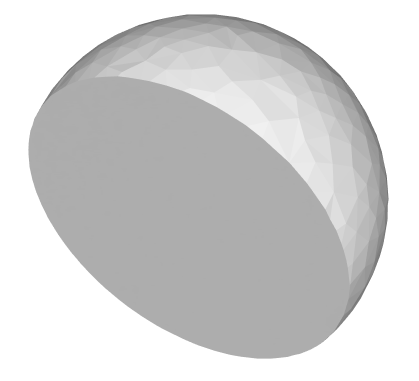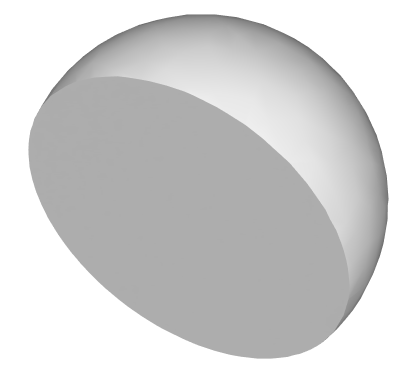Skip To Content

# softenNormals operation

### Synopsis

• softenNormals (angle)

### Parameters

1. anglefloat
For each vertex, use the average of the adjacent face normals. Edges where face normals meet at an angle greater than or equal as specified are shaded as hard edges. Setting an angle of:
• 0 degrees is equivalent to setNormals(hard)
• 30 degrees is equivalent to setNormals(auto)
• 180 degrees is equivalent to setNormals(soft)

Disconnected shapes must be combined and duplicate vertices must be merged in order to compute vertex normals using adjacent face normals.

### Description

The softenNormals operation sets the normals of the current shapes' geometry to the desired mode.

### Examples

 Shaded hemisphere with angle 0 (hard normals).Shaded hemisphere with angle 30 (auto normals).Shaded hemisphere with angle 180 (soft normals).